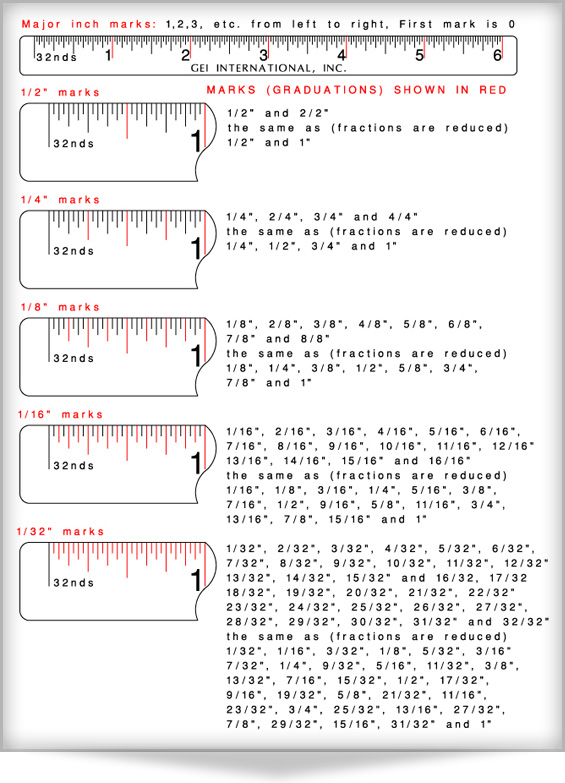# How to Use a Fractional Ruler (Inches)

There are two types of scales commonly used on rulers; Fractional and Decimal. Fractional Rulers have graduations or marks based on fractions, for example 1/2", 1/4" 1/8", 1/16", etc.. Decimal Rulers have graduations or marks which are based on the decimal system such as 0.5, 0.25, 0.1, 0.05, etc..

Most Fractional Rulers are based on the English measuring system where scales are graduated in units of one inch and fractions of an inch.

Fractional Rulers are more difficult to read than Decimal Rulers and mistakes are easily made.

We discuss here, Fractional Rulers based on the English measurement system of Inches.

Fractional rulers can be manufactured with any fraction of an inch as the smallest graduation; however the most common fractional rulers will have some or all of the following fractions of an inch: 3/4", 1/2", 1/4", 1/8", 1/16", 1/32" and 1/64".

The drawing below shows a fractional ruler with a smallest graduation of 1/32" (indicated with a scale legend of 32nds beside the scale) and describes how to measure each fraction of an inch. When writing down a distance measured, normally the whole inches are followed by the fractional part of an inch. For example for a distance of one and three eights of an inch measured, this would normally be written as 1 3/8". Note from the drawing that all fractional measurements are always reduced to their lowest common denominator. 4/8" measured would always be expressed as 1/2", 6/16" as 3/8", 18/32" as 9/16", etc..

Often you will encounter rulers that do not have a legend which identifies what fraction of an inch is utilized on the scale. The ruler below has a legend of 32nds. which indicates that the smallest graduation or mark is 1/32". If a ruler has no legend the smallest fraction of an inch will have to be calculated. Simply count the number of spaces between graduations or marks contained within a single inch. If there are 16 the smallest fraction would be 1/16", if there are 8 the smallest fraction would be 1/8", etc..

CLICK HERE TO VIEW A WIDE VARIETY OF DECIMAL AND FRACTIONAL RULERSManufacturing and Office Location:
GEI International, Inc.
100 Ball Street
East Syracuse, NY 13057 U.S.A.